Telescopes Telescopes and microscopes both use two lenses to produce magnified images. The two lenses of a telescope are called the object lens and the eyepiece. Both are convex (converging) lenses, but the eyepiece has a much shorter focal length. the telescope is illustrated below.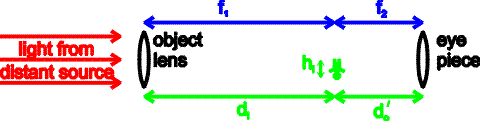The image produced by the object lens lies near the focal point of both lenses. This image then serves as the object for the eye piece. The height, h', of that final image is given by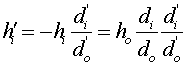The second image which is located a distance di', is far behind the lens. Given that the first image is at the focal point of both lenses, the above equation can be rewritten as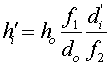Both the distances in the above equation are very large. However, it is angular magnification which is relevant for a telescope, the angle the original object subtends is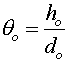, while the angle subtended by the final image is. The ratio of the angles subtended by the original object and the final image is the angular magnification.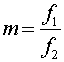The final image seen in a telescope is inverted and appears larger by a factor of the two focal lengths. Since, the first lens should be weak and have a very long focal length, one thus needs a large telescope.